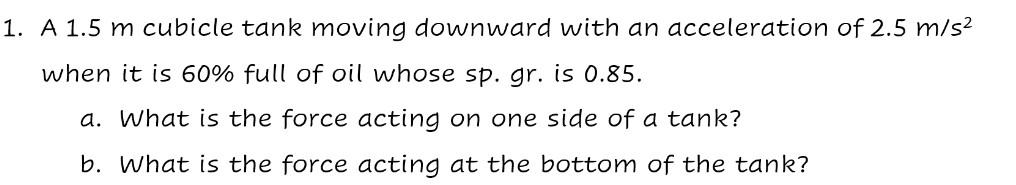# Question 1. A 1.5 m cubicle tank moving downward with an acceleration of 2.5 m/s2 when it is 60% full of oil whose sp. gr. is 0.85. a. What is the force acting on one side of a tank? b. What is the force acting at the bottom of the tank?YXYORU The Asker · Civil EngineeringTranscribed Image Text: 1. A 1.5 m cubicle tank moving downward with an acceleration of 2.5 m/s2 when it is 60% full of oil whose sp. gr. is 0.85. a. What is the force acting on one side of a tank? b. What is the force acting at the bottom of the tank?
More
Transcribed Image Text: 1. A 1.5 m cubicle tank moving downward with an acceleration of 2.5 m/s2 when it is 60% full of oil whose sp. gr. is 0.85. a. What is the force acting on one side of a tank? b. What is the force acting at the bottom of the tank?